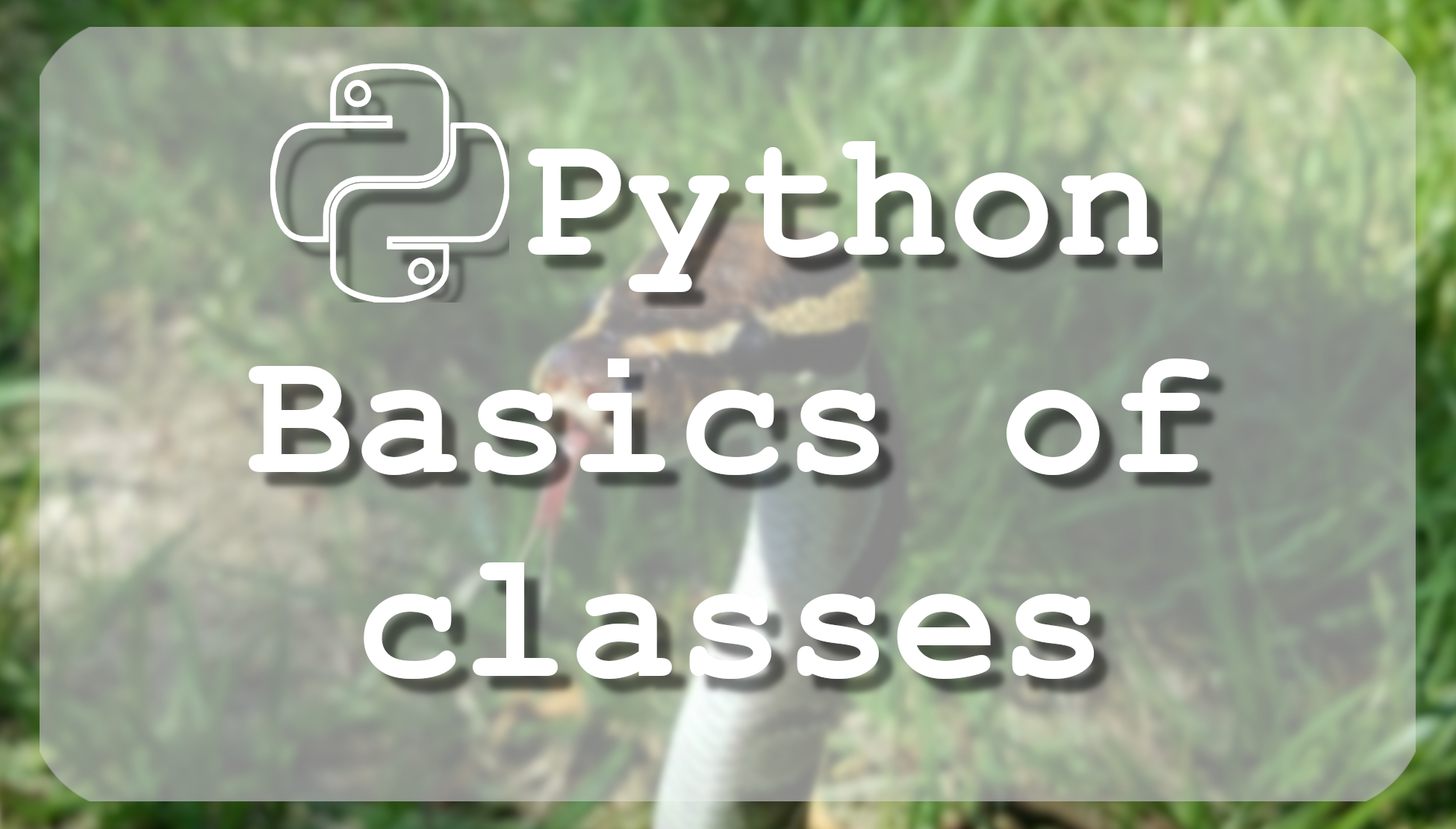# Tutorial - Python 3 basics Classes

## In this tutorial you will learn writing and reading files with python. We will cover basic methods provided how to manipulate files with python.

The Most Advanced Data Science Roadmaps You've Ever Seen! Comes with Thousands of Free Learning Resources and ChatGPT Integration! https://aigents.co/learn/roadmaps/intro

In this tutorial, we are going to cover the very basics of classes in Python. At first, we need to learn to understand and to read classes. If you are just a beginner of programming, at first to understand classes may look quite complicated. For basics, we can think about classes as a group of functions.

Classes are the backbone of Object-Oriented Programming (OOP), if you don't have any previous experience with it, you may want to consult an introductory course on it, or at least some sort of tutorial that you would have a grasp of the basic concepts.

## Simple class syntax:

The class statement creates a new class definition. The name of the class immediately follows the keyword class followed by a colon as follows:

``````class ClassName:
'Optional class documentation string'
class_suite``````
• The class can have a documentation string, which can be accessed via `ClassName.__doc__`.
• Class_suite usually consists of all the component statements defining class members, data attributes, and functions.

Here is a simple class code example for math basics:

``````class calc:

def subtraction(a,b):
sub = a - b
print(sub)

def multiplication(a,b):
mult = a * b
print(mult)

def division(a,b):
div = a / b
print(div)``````

Here are some example lines using with our created calc class:

```>>> calc.addition(66,55)
121
>>> calc.subtraction(66,77)
-11
>>> calc.multiplication(77,88)
6776
>>> calc.division(88,99)
0.8888888888888888
>>> ```

This small basic example will introduce you to classes. Later we will make full classes tutorials for advanced learning.
For example, instead of the print function, you can use return. Then when calling a function you can write for example this: `print(calc.addition(66,55))`.

In this tutorial, we covered the basics of classes.